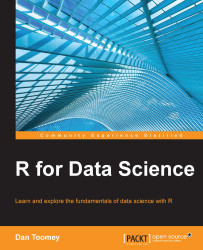•#### R for Data Science#### Overview of this book

R for Data ScienceCreditswww.PacktPub.comPrefaceFree Chapter
Data Mining PatternsData Mining SequencesText MiningData Analysis – Regression AnalysisData Analysis – CorrelationData Analysis – ClusteringData Visualization – R GraphicsData Visualization – PlottingData Visualization – 3DMachine Learning in ActionPredicting Events with Machine LearningSupervised and Unsupervised LearningIndex## Summary

In this chapter, we explored a variety of plotting methods in R. We covered scatter plots, step diagrams, and histograms. We added a regression line and a lowess line to a plot. We used a couple of different tools to plot matrices. We saw a density scatter plot. We used bar graphs. And finally, we generated a word cloud.

In the next chapter, we will cover 3D modeling.